﻿ ﻿GLOSSARY - Quantum: Einstein, Bohr and the Great Debate About the Nature of Reality - Manjit Kumar

# Quantum: Einstein, Bohr and the Great Debate About the Nature of Reality - Manjit Kumar (2009)

### GLOSSARY

Terms in italics have an entry in the glossary.

Alkali elements

Elements such as lithium, sodium and potassium in group one of the periodic table that share the same chemical properties.

Alpha decay

A process of radioactive decay in which the nucleus of an atom emits an alpha particle.

Alpha particle

A subatomic particle consisting of two protons and two neutrons bound together. Emitted during alpha decay, it is identical to the nucleus of a helium atom.

Amplitude

The maximum displacement of a wave or an oscillation that is equal to half the distance from the top of the wave (or oscillation) to the bottom. In quantum mechanics, the amplitude of a process is a number that is linked to the probability of that process occurring.

Angular momentum

A property of a rotating object akin to the momentum of an object moving in a straight line. The angular momentum of an object depends on its mass, its size, and the speed with which it is spinning. One object orbiting another also possesses angular momentum that depends on its mass, the radius of its orbit, and its velocity. In the atomic realm, angular momentum is quantised. It can change only by amounts that are whole-number multiples of Planck's constant divided by 2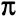.

Atom

The smallest component of an element consisting of a positively-charged nucleus surrounded by a bound system of negatively-charged electrons. Since an atom is neutral, the number of positively-charged protons in the nucleus is equal to the number of electrons.

Atomic number (Z)

The number of protons in the nucleus of an atom. Every element has a unique atomic number. Hydrogen, with a single proton making up its nucleus and one electron orbiting it, has an atomic number of 1. Uranium, with 92 protons and 92 electrons, has an atomic number of 92.

Balmer series

The set of emission or absorption lines in the spectrum of hydrogen caused by the transitions of its electron between the second and higher energy levels.

Bell's inequality

A mathematical condition derived by John Bell in 1964 concerning the degree of correlation of the quantum spins of entangled pairs of particles that has to be satisfied by any local hidden variables theory.

Bell's theorem

A mathematical proof discovered by John Bell in 1964 that any hidden variables theory whose predictions agree with those of quantum mechanics must be non-local. See non-locality.

Beta particle

A fast moving electron ejected from the nucleus of a radioactive element due to the interconversion of protons and neutrons. Faster and more penetrating than alpha particles, it can be stopped by a thin sheet of metal.

Blackbody

A hypothetical, idealised body that absorbs and emits all electromagnetic radiation that strikes it. It can be approximated in the laboratory as a heated box with a pinhole in one of its walls.

Electromagnetic radiation emitted by a blackbody.

Brownian motion

The erratic motion of pollen grains suspended in a fluid first observed, in 1827, by Robert Brown. In 1905 Einstein explained that Brownian motion was due to the random buffeting of the pollen grains by the molecules of the fluid.

Causality

Every cause has an effect.

Classical mechanics

The name given to the physics that originates from Newton's three laws of motion. Also called Newtonian mechanics, in which the properties of particles such as position and momentum are, in principle, simultaneously measurable with unlimited accuracy.

Classical physics

The description applied to all non-quantum physics such as electromagnetism and thermodynamics. Although Einstein's general theory of relativity is regarded by physicists as 'modern' twentieth-century physics, it is nevertheless a 'classical' theory.

Cloud chamber

A device invented by C.T.R. Wilson around 1911 that enables the detection of particles by observing their tracks through a chamber containing saturated vapour.

Collapse of the wave function

According to the Copenhagen interpretation, until it is observed or measured, a microphysical object like an electron does not exist anywhere. Between one measurement and the next it has no existence outside the abstract possibilities of the wave function. It is only when an observation or measurement is made that one of the 'possible' states of the electron becomes its 'actual' state and the probabilities of all the other possibilities become zero. This sudden, discontinuous change in the wave function due to an act of measurement is called the 'collapse of the wave function'.

Commutativity

Two variables A and B are said to commute if A×B=B×A. For example, if A and B are the numbers 5 and 4, then 5×4=4×5. Multiplcation of numbers is commutative, since the order in which they are multiplied makes no difference. If A and B are matrices, then A×B does not necessarily equal B×A. When this happens, A and B are said to be non-commutative.

Complementarity

A principle advocated by Niels Bohr that the wave and particle aspects of light and matter are complementary but exclusive. This dual nature of light and matter is like the two sides of the same coin that can display either face, but not both simultaneously. For example, an experiment can be devised to reveal either the wave properties of light or its particle nature, but not both at the same time.

Complex number

A number written in the form a+ib, where a and b are ordinary real numbers familiar from arithmetic. i is the square root of -1, so that (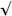-1)2=-1, and b is called the 'imaginary' part of the complex number.

Compton effect

The scattering of photons by atomic electrons discovered by the American physicist Arthur H. Compton in 1923.

Conjugate variables

A pair of dynamical variables such as position and momentum, or energy and time, that are related to one another through the uncertainty principle, are called conjugate variables or conjugate pairs.

Conservation law

A law which states that some physical quantity, such as momentum or energy, is conserved in all physical processes.

Conservation of energy

The principle that energy cannot be created or destroyed, but can only be converted from one form to another. For example, when an apple falls from a tree, its potential energy is converted into kinetic energy.

Copenhagen interpretation

An interpretation of quantum mechanics, whose principal architect Niels Bohr was based in Copenhagen. Over the years there were differences of opinion between Bohr and other leading advocates of the Copenhagen interpretation such as Werner Heisenberg. However, all agreed on its central tenets: Bohr's correspondence principle, Heisenberg's uncertainty principle, Born's probability interpretation of the wave function, Bohr's principle of complementarity, and the collapse of the wave function. There is no quantum reality beyond what is revealed by an act of measurement or observation. Hence it is meaningless to say, for example, that an electron exists somewhere independent of an actual observation. Bohr and his supporters maintained that quantum mechanics was a complete theory, a claim challenged by Einstein.

Correspondence principle

A guiding principle advocated by Niels Bohr in which the laws and equations of quantum physics reduce to those of classical physics under conditions where the impact of Planck's constant is negligible.

De Broglie wavelength

The wavelength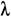of a particle is related to the momentum p of the particle by the relationship=h/p, where h is Planck's constant.

Degrees of freedom

A system is said to have n degrees of freedom if it requires n coordinates to specify each state of the system. Each degree of freedom represents an independent way in which a body can move or a system can change. An object in the everyday world has three degrees of freedom corresponding to the three directions in which it can move - up and down, back and forth, and side to side.

Determinism

In classical mechanics, if the positions and momenta of all the particles in the universe at some instant of time were known, and if all the forces between those particles were also known, then the subsequent state of the universe could in principle be determined. In quantum mechanics it is impossible to specify simultaneously the position and momentum of any particle at any instant. The theory therefore leads to an indeterministic view of the universe, one in which its future cannot be determined in principle. Nor can a particle's.

Diffraction

The spreading out of waves when they pass a sharp edge or through an aperture, such as water waves entering a harbour through a gap in the wall.

Dynamical variables

Quantities used to characterise the state of a particle such as position, momentum, potential energy, and kinetic energy.

Electromagnetic waves differ in the amount of energy they transfer, called electromagnetic radiation. Lower-frequency waves like radio waves emit less electromagnetic radiation than higher-frequency waves such as gamma rays. Electromagnetic waves and electromagnetic radiation are often used interchangeably. See electromagnetic waves and radiation.

Electromagnetic spectrum

The entire range of electromagnetic waves: radio waves, infrared radiation, visible light, ultraviolet radiation, X-rays, and gamma rays.

Electromagnetic waves

Generated by oscillating electric charges, they differ in wavelength and frequency, but all electromagnetic waves have the same speed in empty space, approximately 300,000 kilometres per second. This is the speed of light, and it was the experimental confirmation that light was an electromagnetic wave.

Electromagnetism

Electricity and magnetism were regarded as two distinct phenomena described by their own sets of equations until the latter half of the nineteenth century. Following the experimental work of men like Michael Faraday, James Clerk Maxwell succeeded in developing a theory that unified electricity and magnetism into electromagnetism and described its behaviour in a set of four equations.

Electron

An elementary particle with a negative electric charge that, unlike the proton and the neutron, is not composed of more fundamental component.

Electron volt (eV)

A unit of energy used in atomic, nuclear and particle physics that is about ten-billionth-billionths of a joule (1.6×10-19 joules).

Energy

A physical quantity that can exist in different forms, such as kinetic energy, potential energy, chemical energy, thermal energy, and radiant energy.

Energy levels

The discrete set of allowed internal energy states of an atom corresponding to the different quantum energy states of the atom itself.

Entanglement

A quantum phenomenon in which two or more particles remain inexorably linked no matter how far apart they are.

Entropy

In the nineteenth century, Rudolf Clausius defined entropy as the amount of heat in or out of a body, or a system, divided by the temperature at which the transfer takes place. Entropy is the measure of the disorder of a system; the higher the entropy, the greater the disorder. No physical process that would lead to a decrease in the entropy of an isolated system can occur in nature.

Ether

A hypothetical, invisible medium that was believed to fill all of space and through which light and all other electromagnetic waves were thought to travel.

Exclusion principle

No two electrons can occupy the same quantum state, i.e. have the same set of four quantum numbers.

Fine structure

The splitting of an energy level or spectral line into several distinct components.

Frequency (v)

The number of complete cycles executed by a vibrating or an oscillating system in one second. The frequency of a wave is the number of complete wavelengths that pass a fixed point in one second. The unit of measurement is the hertz (Hz) and is equal to one cycle or wavelength per second.

Gamma rays

Extremely short-wavelength electromagnetic radiation. It is the most penetrating of the three types of radiation emitted by radioactive substances.

Ground state

The lowest energy state that an atom can possess. All other atomic states are called excited states. The lowest energy state of a hydrogen atom corresponds to its electron occupying the lowest energy level. If it occupies any other energy level, the hydrogen atom is in an excited state.

Harmonic oscillator

A vibrating or oscillating system whose frequency of vibration or oscillation does not depend on the amplitude.

Hidden variables

An interpretation of quantum mechanics based on the belief that the theory is incomplete and that there is an underlying layer of reality that contains additional information about the quantum world. This extra information is in the form of the hidden variables, unseen but real physical quantities. The identification of these hidden variables would lead to exact predictions for the outcomes of measurements and not just probabilities of obtaining certain results. Its adherents believe that it would restore a reality that exists independently of observation, denied by the Copenhagen interpretation.

Electromagnetic radiation with wavelengths longer than visible red light.

Interference

This is a characteristic phenomenon of wave motion in which two waves interact. Where two wave troughs or crests meet, they coalesce to produce a new, bigger trough or crest; this is known as constructive interference. But where a trough meets a crest or vice versa, they cancel each other out, a process called destructive interference.

Isotopes

Different forms of the same element that have the same number of protons in the nucleus, i.e. that share the same atomic number, but each having a different number of neutrons. For example, there are three forms of hydrogen with their nuclei containing zero, one, and two neutrons respectively. All three have similar chemical properties but different masses.

Joule

A unit of energy used in classical physics. A 100-watt light bulb converts 100 joules of electrical energy per second into heat and light.

Kinetic energy

Energy associated with the motion of an object. A stationary object, planet or particle has no kinetic energy.

Light

The human eye can detect only a small portion of all electromagnetic waves. These visible wavelengths of the electromagnetic spectrum range between 400nm (violet) and 700nm (red). White light is made up of red, orange, yellow, green, blue, indigo, and violet light. When a beam of white light is passed through a glass prism, these different strands of light are unpicked and form a rainbow band of colours called a continuum or continuous spectrum.

Light-quanta

The name first used by Einstein in 1905 to describe particles of light, later renamed photons.

Locality

The requirement that a cause and its effects occur at the same place, that there is no action at a distance. If an event A is the cause of another at B, there must be enough time between the two to allow a signal travelling at the speed of light from A to reach B. Any theory which has locality is called local. See non-locality.

Matrices

Arrays of numbers (or other elements such as variables) with their own rules of algebra, matrices are extremely useful for expressing information about a physical system. An n×n square matrix has n columns and n rows.

Matrix mechanics

A version of quantum mechanics discovered by Heisenberg in 1925 and then developed in conjunction with Max Born and Pascual Jordan.

Matter wave

When a particle behaves as though it has a wave character, the wave representing it is called a matter wave or a de Broglie wave. See deBroglie wavelength.

Maxwell's equations

A set of four equations derived by James Clerk Maxwell in 1864 that unified and described the disparate phenomena of electricity and magnetism as a single entity - electromagnetism.

Momentum (p)

A physical property of an object that is equivalent to its mass times its velocity.

Nanometre (nm)

One nanometre is equal to one billionth of a metre.

Neutron

An uncharged particle that is similar in mass to a proton.

Non-locality

An influence is allowed to pass between two systems or particles instantaneously, exceeding the limit set by the speed of light, so that a cause at one place can produce an immediate effect at some distant location. Any theory that allows non-locality is called non-local. See locality.

Nucleus

The positively-charged mass at the heart of an atom. Initially believed to be made up only of protons, but later found to include neutrons. It contains virtually the entire mass of an atom but occupies a tiny fraction of its volume. Discovered in 1911 by Ernest Rutherford and his co-workers at Manchester University.

Observable

Any dynamical variable of a system or object that can, in principle, be measured. For example, the position, momentum, and kinetic energy of an electron are all observables.

Period

The time it takes for a single wavelength to pass a fixed point, and also the time required to complete one cycle of an oscillation or vibration. The period is inversely proportional to the frequency of a wave, vibration, or oscillation.

Periodic table

The arrangement of the elements according to their atomic number into rows and columns that displays their recurring chemical properties.

Photoelectric effect

The emission of electrons from a given metal surface when electromagnetic radiation above a certain minimum frequency (wavelength) strikes it.

Photon

The quantum of light characterised by the energy E=hv and momentum p=h/where v andare the frequency and wavelength of the radiation. The name was introduced in 1926 by the American chemist Gilbert Lewis. See light-quanta.

Planck's constant (h)

A fundamental constant of nature with a value of 6.626×10-34 joule-seconds that lies at the heart of quantum physics. Because Planck's constant is not zero, it is responsible for chopping up, quantising, energy and other physical quantities in the atomic realm.

Potential energy

The energy that an object or system has by virtue of its position or state. For example, the height of an object above the earth's surface determines its gravitational potential energy.

Probability interpretation

The interpretation suggested by Max Born that the wave function allowed only the probability of finding a particle at a particular location to be calculated. It is part and parcel of the idea that quantum mechanics can generate only the relative probabilities of obtaining certain results from the measurement of an observable and cannot predict which specific result will be obtained on a given occasion.

Proton

A particle contained in the nucleus of an atom that carries a positive charge equal and opposite to that on an electron and that has a mass some 2,000 times that of the electron's.

Quantised

Any physical quantity that can only have certain discrete values is quantised. An atom has only certain discrete energy levels and its energy is therefore quantised. The spin of an electron is quantised since it can only be either +½ (spin up) or -½ (spin down).

QUANTUM

A term introduced by Max Planck in 1900 to describe the indivisible packets of energy that an oscillator could emit or absorb in his model as he tried to derive an equation that reproduced the distribution of blackbody radiation. A quantum of energy (E) comes in various sizes determined by E=hv, where h is Planck's constant and v is the frequency of the radiation. 'Quantum', more properly 'quantised', can be applied to any physical property of a microphysical system or object that is discontinuous, that can change only by discrete units.

Quantum jump

Also known as a quantum leap, it is the transition of an electron between two energy levels inside an atom or molecule due to the emission or absorption of a photon.

Quantum mechanics

The theory of physics of the atomic and subatomic realm that replaced the ad hoc mixture of classical mechanics and quantum ideas that emerged between 1900 and 1925. Although dissimilar, Heisenberg's matrix mechanicsand Schrödinger's wave mechanics are two mathematically equivalent representations of quantum mechanics.

Quantum number

Numbers that specify quantised physical quantities such as energy, quantum spin or angular momentum. For example, the quantised energy levels of a hydrogen atom are denoted by a set of numbers beginning with n=1 for the ground state, where n is the principal quantum number.

Quantum spin

A fundamental property of particles with no direct counter-part in classical physics. Any picturesque comparison of a 'spinning' electron to a spinning top is merely a poor aid that fails to capture the essence of this quantumconcept. The quantum spin of a particle cannot be explained in terms of classical rotation since it can only have certain values that are equal to either a whole number or half a whole number multiplied by Planck's constant h divided by 2(h, a quantity called h-bar). Quantum spin is said to be either up (clockwise) or down (anti-clockwise) with respect to the direction of measurement.

The emission of energy or particles. Examples include electromagnetic radiation, thermal radiation and radioactivity.

When an unstable atomic nucleus spontaneously disintegrates to acquire a more stable configuration by emitting alpha, beta or gamma radiation, the process is called radioactivity or radioactive decay.

Realism

The philosophical worldview which maintains that there exists a reality 'out there' independent of an observer. For a realist, the moon exists when no one is looking at it.

Relativity, general

Einstein's theory of gravitation in which the gravitational force is explained as a distortion of space-time.

Relativity, special

Einstein's 1905 theory of space-time in which the speed of light remains the same for all observers however fast they are moving. It is called 'special' because it does not describe objects that are accelerating, or gravity.

Scattering

The deflection of one particle by another.

Schrödinger's cat

A thought experiment devised by Erwin Schrödinger in which, according to the rules of quantum mechanics, a cat exists in a superposition of alive and dead states until it is observed.

Schrödinger's equation

The fundamental equation of the wave mechanics version of quantum mechanics that governs the behaviour of a particle or the evolution of a physical system by encoding how its wave function varies with time.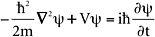where m is the mass of the particle,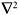is a mathematical entity called the 'del-squared operator' which is responsible for tracking how the wave function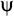changes from place to place, V captures the forces acting on the particle, i is the square root of -1,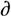/t describes how the wave functionchanges in time, and h is Planck's constant h divided by 2and is pronounced 'h-bar'. There is another form of the equation that gives a snapshot in time and is called the time-independent Schrödinger equation.

Spectral energy distribution of blackbody radiation

At any given temperature, it is the intensity of electromagnetic radiation emitted by a blackbody at each wavelength (or frequency). Also known simply as the blackbody spectrum.

Spectral lines

The pattern of coloured lines of light on a black background is called an emission spectrum. A series of black lines on a coloured background is called an absorption spectrum. Each element has a unique set of both emission and absorption spectral lines produced respectively by the emission and absorption of photons as electrons within the atoms of the element jump between different energy levels.

Spectroscopy

The area of physics concerned with analysing and studying absorption and emission spectra.

Spontaneous emission

The spontaneous emission of a photon as an atom makes the transition from an excited state to a lower energy state.

Stark effect

The splitting of spectral lines when atoms are placed in an electric field.

Stimulated emission

When an incident photon is not absorbed by an excited atom, but 'stimulates' it to emit a second photon of the same frequency.

Superposition

A quantum state composed of two or more other states. Such a state has certain probabilities for exhibiting the properties of the states out of which it is composed. See Schrödinger's cat.

Thermodynamics

Commonly described as the physics of the transformation of heat into and from other forms of energy.

Thermodynamics, the first law

The internal energy of an isolated system is a constant. Or equivalently, energy cannot be created or destroyed - the principle of the conservation of energy.

Thermodynamics, the second law

Heat does not flow spontaneously from cold to hot objects. Or equivalently, since there are different formulations of the law, the entropy of a closed system cannot decrease.

Thought experiment

An idealised, imaginary experiment conceived as a means to test the consistency or limits of a physical theory or concept.

Ultraviolet catastrophe

Classical physics distributes an infinite amount of energy among the high frequencies of blackbody radiation. This so-called ultraviolet catastrophe predicted by classical theory does not occur in nature.

Ultraviolet light

Electromagnetic radiation with wavelengths shorter than those of visible violet light.

Uncertainty principle

The principle discovered by Werner Heisenberg in 1927 that it is not possible to measure simultaneously certain pairs of observables - such as position and momentum, energy and time - with a degree of accuracy that exceeds a limit expressed in terms of Planck's constant h.

Velocity

The speed of an object in a given direction.

Wave function ()

A mathematical function associated with the wave properties of a system or particle. The wave function represents everything that can be known about the state of a physical system or particle in quantum mechanics. For example, using the wave function of the hydrogen atom it is possible to calculate the probability of finding its electron at a certain point around the nucleus. See probability interpretation and Schrödinger's equation.

Wave mechanics

A version of quantum mechanics developed in 1926 by Erwin Schrödinger.

Wave packet

A superposition of many different waves that cancel each other out everywhere except within a small confined region of space, allowing the representation of a particle.

Wave-particle duality

Electrons and photons, matter and radiation, may behave either like waves or like particles depending upon the experiment performed.

Wavelength ()

The distance between two successive peaks or troughs of a wave. The wavelength of electromagnetic radiation determines which part of the electromagnetic spectrum it belongs to.

Wien's displacement law

Wilhelm Wien discovered in 1893 that as the temperature of a blackbody increases, the wavelength at which it emits the greatest intensity of radiation shifts to ever-shorter wavelengths.

Wien's distribution law

A formula discovered by Wilhelm Wien in 1896 that described the distribution of blackbody radiation in accordance with the experimental data then available.

X-rays

The radiation discovered by Wilhelm Röntgen in 1895 for which he was awarded the first Nobel Prize for physics in 1901. X-rays were later identified as electromagnetic waves of extremely short wavelength, emitted when very fast-moving electrons strike a target.

Zeeman effect

The splitting of spectral lines when atoms are placed in a magnetic field.

﻿